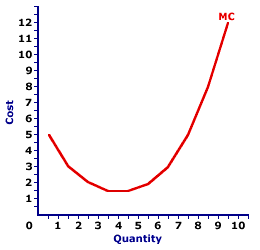Wednesday  February 1, 2023
 AmosWEB means Economics with a Touch of Whimsy!CAPITAL ACCOUNT SURPLUS: An imbalance in a nation's balance of payments capital account in which payments received by the country for selling domestic assets exceed payments made by the country for purchasing foreign assets. In other words, investment by the domestic economy in foreign assets is greater than foreign investment in domestic assets. This is generally a desireable situation for a domestic economy. However, in the wacky world of international economics, a capital account surplus is often balanced by a current account deficit, which is not generally considered a desireable situation. If, however, the current account does not balance out the capital account, then a capital account surplus contributes to a balance of payments surplus.MARGINAL COST CURVE:

A curve that graphically represents the relation between the marginal cost incurred by a firm in the short-run product of a good or service and the quantity of output produced. This curve is constructed to capture the relation between marginal cost and the level of output, holding other variables like technology and resource prices constant. Three related curves are average total cost curve, average variable cost curve, and average fixed cost curve.
The marginal cost curve, the graphical relation between marginal cost and output, is U-shaped. Marginal cost is relatively high at small quantities of output, then as production increases, it declines, reaches a minimum value, then rises once again.

This U shape is directly attributable to increasing, then decreasing marginal returns (and the law of diminishing marginal returns). As marginal product (and marginal returns) increases for relatively small output quantities, marginal cost declines. Then as marginal product (and marginal returns) decreases with the law of diminishing marginal returns for relatively large output quantities, marginal cost increases.

Marginal Cost CurveThe graph presented at the right is the marginal cost curve for the short-run production of Wacky Willy Stuffed Amigos (those cute and cuddly scorpions and turtles). The quantity of Stuffed Amigos production, measured on the horizontal axis, ranges from 0 to 10 and the marginal cost incurred in the production of Stuffed Amigos, measured on the vertical axis, starts at \$5, declines to \$1.50, then rises again to \$12.

The marginal cost curve is U-shaped. For the first 4 Stuffed Amigos, marginal cost declines from \$5 to a low of \$1.50. However, for the production beyond 6 Stuffed Amigos, marginal cost increases. The source of this U-shaped marginal cost curve rests with increasing and decreasing marginal returns. In fact, the negatively-sloping portion of the marginal cost curve coincides exactly with increasing marginal returns in production Stage I. The positively-sloping portion of the marginal cost curve coincides exactly with decreasing marginal returns in production Stage II.

The marginal cost curve takes center stage in the analysis of a firm's short-run production. In particular, a profit-maximizing firm equates the marginal revenue received from selling a good with the marginal cost of producing it. For a firm operating under perfect competition, its marginal cost curve becomes its supply curve. The marginal cost curve, because it measures the incremental opportunity cost of producing one more unit of a good plays, an important role in analyzing the efficient allocation of resources.

 <= MARGINAL COST AND MARGINAL PRODUCT MARGINAL COST OF SEARCH =>Recommended Citation:

MARGINAL COST CURVE, AmosWEB Encyclonomic WEB*pedia, http://www.AmosWEB.com, AmosWEB LLC, 2000-2023. [Accessed: February 1, 2023].

Check Out These Related Terms...

Or For A Little Background...

And For Further Study...
Search Again?WHITE GULLIBON[What's This?] Today, you are likely to spend a great deal of time looking for a downtown retail store hoping to buy either a birthday gift for your uncle or a pair of red and purple designer socks. Be on the lookout for telephone calls from former employers.Your Complete ScopeFrancis Bacon (1561-1626), a champion of the scientific method, died when he caught a severe cold while attempting to preserve a chicken by filling it with snow."For a writer, published works are like fallen flowers, but the expected new work is like a calyx waiting to blossom."-- Cao Yu, PlaywrightMPSMarginal Propensity to SaveA PEDestrian's Guide Xtra CreditTell us what you think about AmosWEB. Like what you see? Have suggestions for improvements? Let us know. Click the User Feedback link.| | | | | | | | | | |
| | | |

Thanks for visiting AmosWEB